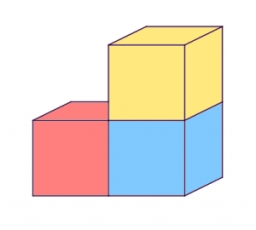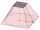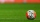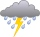# Surface of the cube

Find the surface of the cube that has volume
1/1m3
2/0.001 m3
3/8000 mm3

Result

S1 =  6 m2
S2 =  0.06 m2
S3 =  2400 mm2

#### Solution:

$V_{1}=1 \ \text{m}^3 \ \\ V=a^3 \ \\ a_{1}=\sqrt{ V_{1}}=\sqrt{ 1 }=1 \ \text{m} \ \\ S_{1}=6 \cdot \ a_{1}^2=6 \cdot \ 1^2=6 \ \text{m}^2$
$V_{2}=0.001 \ \text{m}^3 \ \\ a_{2}=\sqrt{ V_{2}}=\sqrt{ 0.001 }=\dfrac{ 1 }{ 10 }=0.1 \ \text{m} \ \\ S_{2}=6 \cdot \ a_{2}^2=6 \cdot \ 0.1^2=\dfrac{ 3 }{ 50 }=0.06 \ \text{m}^2$
$V_{3}=8000 \ \text{mm}^3 \ \\ \ \\ a_{3}=\sqrt{ V_{3}}=\sqrt{ 8000 }=20 \ \text{mm} \ \\ S_{3}=6 \cdot \ a_{3}^2=6 \cdot \ 20^2=2400 \ \text{mm}^2$Our examples were largely sent or created by pupils and students themselves. Therefore, we would be pleased if you could send us any errors you found, spelling mistakes, or rephasing the example. Thank you!

Leave us a comment of this math problem and its solution (i.e. if it is still somewhat unclear...):Be the first to comment!Tips to related online calculators
Do you know the volume and unit volume, and want to convert volume units?

## Next similar math problems:

1. Magnified cubeIf the lengths of the edges of the cube are extended by 5 cm, its volume will increase by 485 cm3. Determine the surface of both the original and the magnified cube.
2. Volume and surface areaFind the volume and surface of a wooden block with dimensions: a = 8 cm, b = 10 cm, c = 16 cm.The surface of the regular quadrilateral prism is 8800 cm2, the base edge is 20 cm long. Calculate the volume of the prism
4. The water tankThe water tank has the shape of a sphere with a radius of 2 m. How many liters of water will fit in the tank? How many kilograms of paint do we need to paint the tank, if we paint with 1 kg of paint 10 m2?
5. Pentagonal prismThe regular pentagonal prism is 10 cm high. The radius of the circle of the described base is 8 cm. Calculate the volume and surface area of the prism.
6. Cone from cubeThe largest possible cone was turned from a 20 cm high wooden cube. Calculate its weight if you know that the density of wood was 850 kg/m3
7. Oak trunkCalculate in tonnes the approximate weight of a cylindrical oak trunk with a diameter of 66 cm and a length of 4 m, knowing that the density of the wood was 800 kg/m³.
8. Copper Cu wireCopper wire with a diameter of 1 mm and a weight of 350 g is wound on a spool. Calculate its length if the copper density is p = 8.9 g/cm cubic.
9. AL wireWhat is the weight of an aluminum wire 250 m long with a diameter of 2 mm, if the density of aluminum is p = 2700 kg/m cubic. Determine to the nearest gram.
10. Runcated pyramid teapotThe 35 cm high teapot has the shape of a truncated pyramid with the length of the edge of the lower square base a=50 cm and with the edges of the rectangular base b: 20 cm and c: 30 cm. How many liters of water will fit in the teapot?
11. What is bigger?Which ball has a larger volume: a football with a circumference of 66 cm or a volleyball with a diameter of 20 cm?
12. The conicalThe conical candle has a base diameter of 20 cm and a side of 30 cm. How much dm ^ 3 of wax was needed to make it?
13. Water tank300hl of water was filled into the tank 12 m long and 6 m wide. How high does it reach?
14. Tunnel boringHow much material did they dig when cutting the 400m long tunnel? The content of the circular segment, which is the cross section of the tunnel is 62m2.
15. RainGarden shape of a rectangle measuring 15 m and 20 m rained water up to 3 mm. How many liters of water rained on the garden?
16. CannonballsOf the three cannonballs with a diameter of 16 cm, which landed in the castle courtyard during the battle, the castle blacksmith cast balls with a diameter of 10 cm, which fit into the cannons placed on the walls. How many cannonballs did the blacksmith c
17. Water mixingWe have 520 ml of hot water and 640 ml of water at 48°C. What is the temperature of approximately hot water when the resulting mixture has a temperature of 65°C?